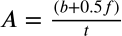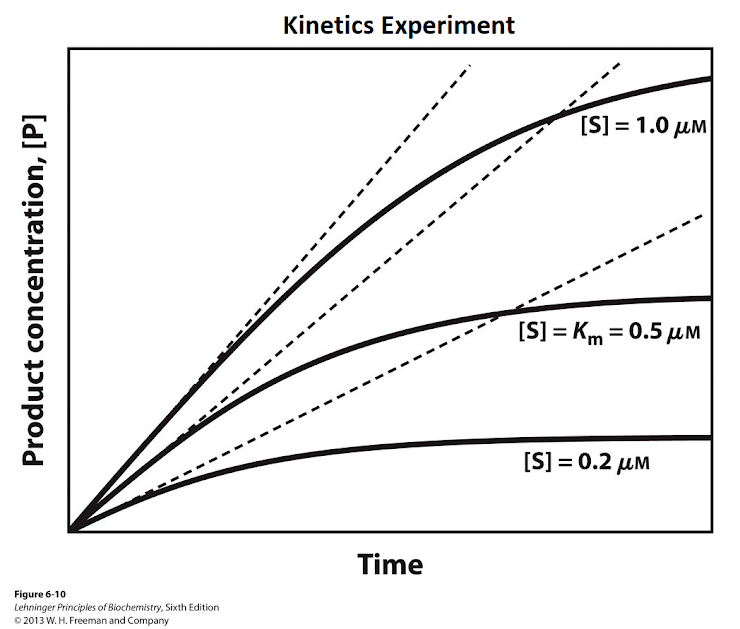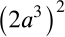Placement Pre-Test for CVC Honors Chemistry
CVC Honors Chemistry is a very rigorous and intense course that requires excellent math and word-problem solving skills. This pre-test is not mandatory in order to register for Honors Chemistry but having your student complete the test could be helpful in determining whether his/her math skills are on par with what is needed to be successful in the course. A student should be able to complete the test in the allotted time and following the directions of each section and have at least 11/13 correct at the end of the pre-test. ~with thanks to the RSC and High Point University
MULTIPLE CHOICE SECTION
This section of the pre-test should be completed WITHOUT the use of a calculator. It should take the student no longer than 15 minutes to complete this section.
1. A bag of apples contains 6 green apples and 8 red apples. What is the approximate percentage of green apples? *
1 point
2. Which of the following values is consistent with 9.17%? *
1 point
3. A reaction requires 4 min 15 sec to complete. Which of the following is a correct representation of that time period? *
1 point
4. How many significant figures does the following number have: 1032.200 *
1 point
5. Considering significant digits, what is the correct answer to this problem? 101.22 + 222.3 = *
1 point
6. Given the following equation, what does f equal? *
1 point7. A "cubit" is an ancient measurement now defined as: 1.0 cubit (c) = 45.72 cm. Which of the following computations should you use to convert a measurement of 0.33 c to centimeters? *
1 point
8. If 6p-3 = 8p-9, then p= *
1 point
9. Find the values of x and y that satisfy both of these equations simultaneously: 2x+y=2 and 2x-y=6 *
1 point
10. Using the graph below, which of the following is correct language to use about this graph? *
1 point11. What is the value of the expression below? *
1 point12. Which of the following shows 0.02156 in scientific notation? *
1 point